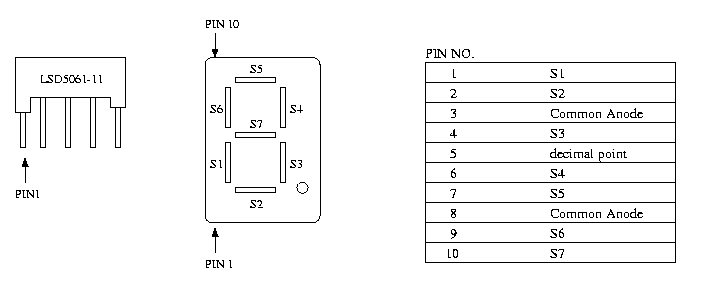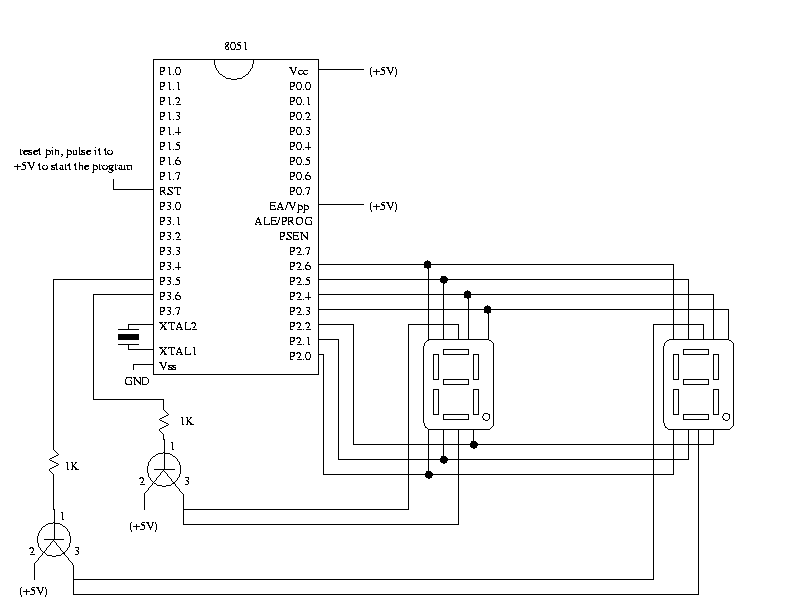Decimal Counter and Multiplexing the Output

Introduction

The purpose of this lab is to implement a decimal counter which counts from 0 to 99. You will have to write a C program for the 80X51 micro-controller. You will then compile your C program using C51 compiler and burn it unto an 8051 stand alone chip. You will also have to do some wiring in this lab. The 8051 chip requires some connections to function properly, and the 7-segment displays need to be wired to the 8051. Schematics are provided below.

Seven Segment Display

Before you can write your C program, you have to understand how the 7-segment displays work. The 7-segment displays used in the lab are the LSD5061-11 display. Each of the segment corresponds to a pin (see below for the pinout). In order to light up a particular segment, it's pin must be set to 0V. Since these pins are connected to the 8051, we simply set the corresponding pin on the 8051 to '0'. To turn a segment off, the pin must be set to 5V. This is done by setting the corresponding pin on the 8051 to "1".

Instead of going through each of the seven pins and setting them to '1' or '0' each time we want to display a number, we will use a lookup table unsigned char LookupTable = { }. The location of the entry in the table corresponds to the correct pin settings to display that number. Simply, LookupTable returns the correct pin settings to display a "0" on the 7-segment display.

Now we have to figure out the correct entries into the table. We know that in order to display a "0" on the 7-segment display we need to turn on segments s1, s2, s3, s4, s5, and s6. To turn a segment on, we set the corresponding pin to "0". Segment s7 will need to be turned off. To turn off this segment we set the corresponding pin to "1". Therefore, the pins will need to be assigned the following values:

P2^0 = 0;
P2^1 = 0;
P2^2 = 0;
P2^3 = 0;
P2^4 = 0;
P2^5 = 0;
P2^6 = 0;
P2^7 = 1;
P2^8 = 1; //don't care what this value is since it is not used We set it to 1 because the 8051 pins are by default 1

The bit pattern desired is 1100 0000 (remeber that pin 8 is the highest bit). We then convert the values into hexadecimal 1100 -> C and 0000 -> 0. We fill in the table with this value 0xC0. The "0x" is needed before the actual value to indicate to the compiler that it is a hexadecimal value. Now when we call LookupTable it will return the proper configuration to display a "0" on the 7-segment display. You will need to fill in the rest of the values (1-9). LookupTable will indicate an error has occured, at this location segment s7 will be on, the remaining segments will be off. (If you want, you can also look at the 7-segment display datasheet.)Multiplexing the Output

We have two 7-segment displays connected to the same port on the 8051. Because the circuit is connected in this way we have to multiplex the output. This means that we will turn on the 7-segment display corresponding to the one's place, turn off the ten's place 7-segment display, and configure the output port to display the one's value. Then we will turn on the 7-segment display corresponding to the tens place, turn off the one's place 7-segment display, and configure the output port to display the ten's place. If we do this fast enough, we will not be able to notice that one of the 7-segment displays is off and one of the 7-segment displays is on. It will look as if both segments are being handled seperately.

The next question is how we are supposed to turn the 7-segment displays off and on. You will notice that there are two pins on each of the the 7-segment displays which correspond to common anode. If we set these pins to +5V the 7-segment display is on. If we set these pins to 0V the 7-segment display if off. The two common anode pins corresponding to the ten's place display are connected to P3^6. If we want to turn the ten's place display on we simply set P3^6 to 1. If we want to turn the ten's place display off we set P3^6 to 0. The same applies for the one's place display connected to P3^5. To turn it on we set P3^5 to 1 and to turn it off we set P3^5 to 0. The problem we then encounter is that the 8051 cannot drive components. Althougth we set a particular pin to 1, the 8051 does not provide the +5V needed by the 7-segment display. To remedy this we must use transistors. The schematic below shows how to connect these.

Assignment

In this lab :

• You will implement a decimal counter using the C programming language for the 8051 micro-controller and display the count (0 to 99) on two 7-segment displays.
• You will then burn your program on the 8051.
• Make necessary connections the 8051 chip and the two 7-segment displays.
• Verify the correctness of your circuit.

Apparatus Required

1. 1K resistors (1)
2. MJE 3055T transistors (2)
3. LSD5061-11 7-segment display (2)
4. 5V power supply
5. 8051 chip
6. 12MHz crystal (clock)

SchematicProgram

```
/* main.c */

/* decimal counter with multiplexed output */

#pragma SMALL DB
#include

/* P0, P1, P2 and P3 are predefined port names and are bit addressable */

unsigned char SetDisplay(unsigned char value){

unsigned char LookupTable = { 0xC0, ... };

/* check to see if "value" is in bounds */
if( ) {
/* return appropriate value */
}
else {
/* return appropriate value */
}
}

/* Delay function */
void delay() {
int i;

for(i=0; i<1000; i++){
i = i + 0;
}
}

void main(void){
unsigned char count = 0;    /* holds value to be displayed */
unsigned long timer = 0;
int turn = 1;               /* indicates if we display the ones
place or the tens place */

while(1) {
if(turn){
/* configure P3 to turn on the one's place display */
/* display the one's value */
turn = 0;
delay();

}
else{
/* configure P3 to turn on the ten's place display */
/* display the ten's value */
turn = 1;
delay();

}

if(timer == 100){
/* update the counter value */
timer=0;
}

timer++;
}
}
```

Procedure

Hardware

1. Wire up the circuit as shown in the schematic.
2. Pinouts are also available:
Software
1. Map your network drive to P:\\Peart\cs122
2. Run the batch file cs122.bat
3. Create a directory for your program on the C: drive (i.e. C:\Temp\counter)
4. Using a text editor (i.e. notepad, etc...) type in the program provided and add any necessary code to your program.
5. Compile your program to generate object file count.obj
```	   c51 count.c
```
7. ```	   bl51 count.obj to count.omf
```
8. Create a hex file, count.hex, used to burn your program unto the 8051 chip
```           oh51 count.omf
```
9. Copy your hex file to a floppy
```           copy count.hex A:\count.hex
```
Burning A Chip - Programmer: MP-51
1. Place the chip to be programmed on the slot making sure that the correct side is up
2. Use the arrow keys on the keyboard to choose options
3. Choose Type -> 51Controller -> PC89C52U
4. Choose File -> Load and enter the pathname of your hex file (i.e. A:\counter.hex)
5. Choose Command -> Program -> DeviceMemory -> Program Device Memory
6. The chip will be programmed. Remove it from the chip from the socket. It is now ready to be plugged into your board.